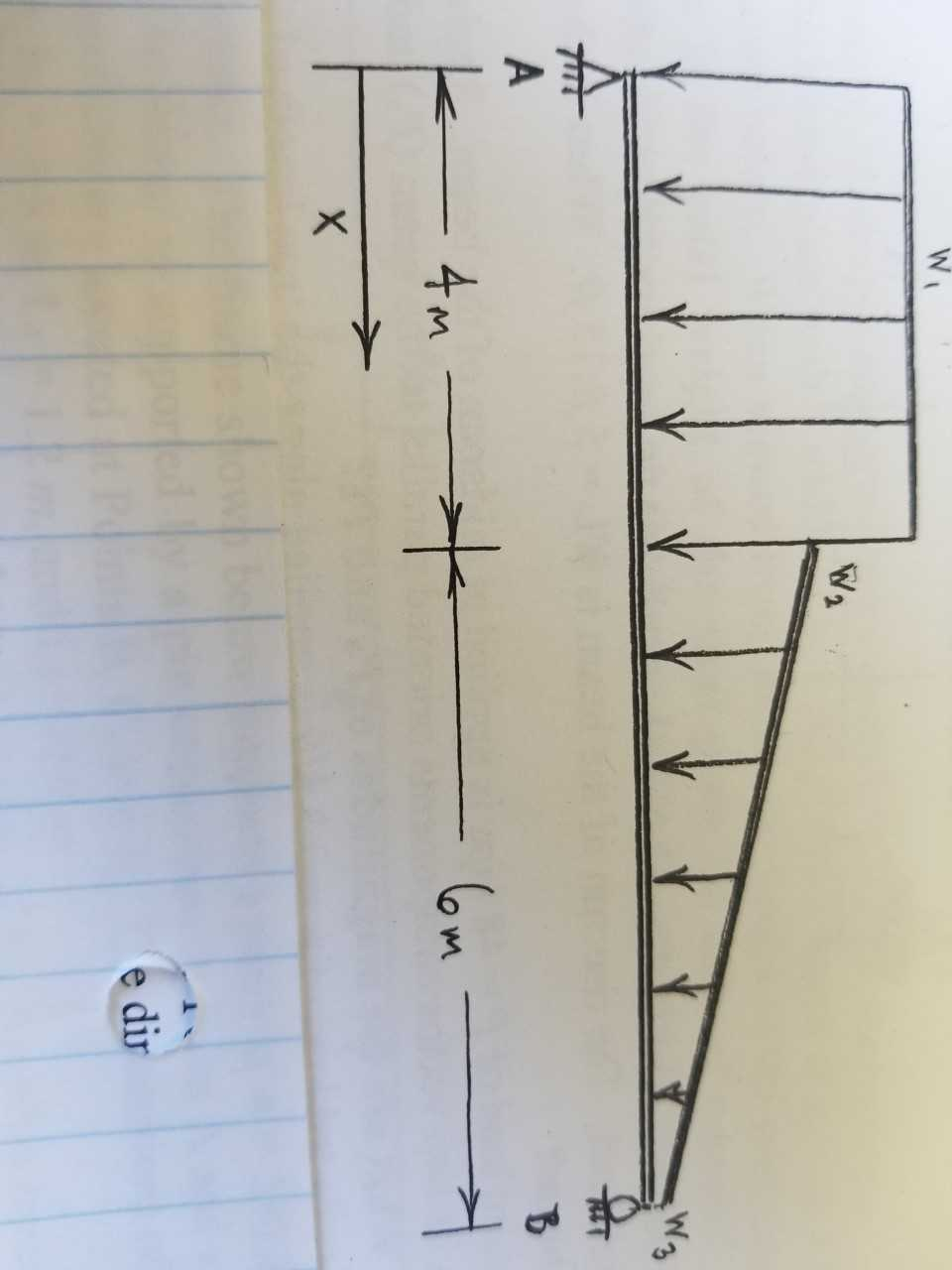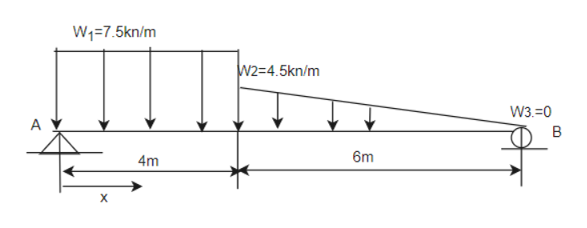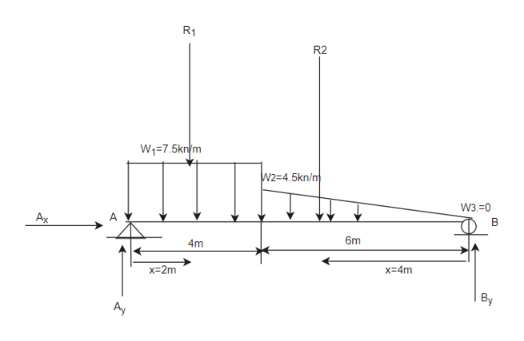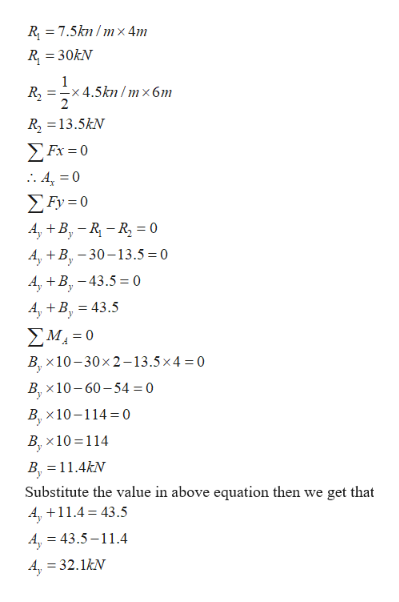A4momdir

Question

The beam is supported by a pin connection at A and a roller at B. Beam AB supports a uniformly distributed load of 7.5 kN/m over the first 4 meters (x=0 to x=4) and supports a distributed load that decreases from w2=4.5kN/m to w3=0kN/m. Over all beam length (x) is 10 meters. Neglecting the self-weight of member AB, find"

• The reaction forces at A and B.
• The internal shear and moment at mid-span (x=5m)
• The equations for internal shear, V(x), and internal moment M(x), as a function of  location (x) in the region for x between 0 and 4m of the beam.help_outlineImage TranscriptioncloseA 4m om dir fullscreen
Step 1

Given:

The beam ishelp_outlineImage TranscriptioncloseW1-7.5kn/m W2=4.5kn/m W3-0 A 6m 4m X fullscreen
Step 2

Calculation:

1. The reaction forces at A and B

To calculate the support reactions replace the distributed load into equivalent concentrated loads.

Then,help_outlineImage TranscriptioncloseR1 R2 W1=7.5kn/m W2-4.5kn/m W3-0 Ax B 6m 4m x-2m x-4m By A fullscreen
Step 3help_outlineImage Transcriptionclose7.5kn /mx 4m R R 30kN =-x4.5kn /m x 6m R 13.5kN Σκ - 4 0 ΣΕν-0 4,B-RR 0 A, +B-30-13.5 = 0 4, +B43.5 0 A, B 43.5 ΣΜ.-0 Bx10-30x2-13.5 x 4 = 0 x10-60-54 0 B, x10-114 0 B x10 114 B, 11.4kN Substitute the value in above equation then we get that A,11.4 43.5 A, =43.5-11.4 A 32.1kN fullscreen

Want to see the full answer?

See Solution

Want to see this answer and more?

Our solutions are written by experts, many with advanced degrees, and available 24/7

See Solution
Tagged in

Solid Mechanics